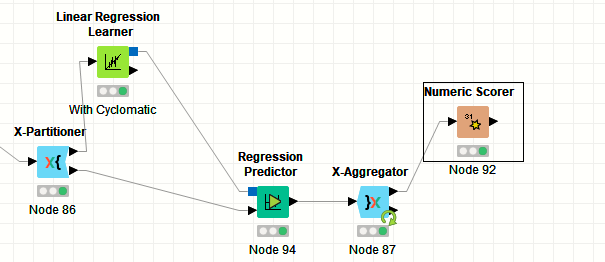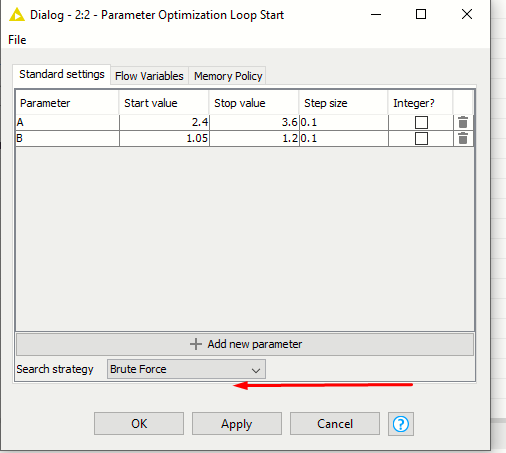# How to optimize the two variable's values in linear regression

How to optimize the two (A, B) variables values using linear regression.I have three values in A variable the range is (2.4, 3.0, 3.6) and B (1.05, 1.12,1.20)
I know the two nodes (Parameters Optimization Loop) available in knime but I am not an expert in KNIME so I am confused that how can I use these two nodes (Parameters Optimization Loop).
Here is the screenshot of the values in my dataset.

Hi,
what do you mean by “optimizing A and B”? If you want to do Linear Regression, you need features (independent variables, X) and a dependent variable (y) that you want to predict based on the features. What are the features in your case and what is the dependent variable? A Parameters Optimization Loop is used to optimize hyperparameters of a given learner, but in the case of linear regression there are not really any hyperparameters you need to optimize. Please clarify your goal of this analysis.
Kind regards
Alexander

1 Like

These are the independent variables

• Files (Number (integer))
• Statements (Number (integer))
• Calls (Number (integer))
• Classes (Number (integer))
• Total Complexity (Number (integer))
• KLOC (Number (double))

and this is the target variable Effort
We use Effort = A(KLOC) ^ B to create the effort column/ dependent variable.
I have already described the A and B values.
We check the KLOC to select the A and B values

KLOC < 50 (Use A=3.2, B=1.05)
KLOC > 50 && KLOC < 300 (Uuse A=3 , B=1.12)
KLOC > 300 (Use A=2.8, B=1.20)

Actually it’s a Cocomo formula we use Cocomo model to get the effort for software.

Hi,
in this case, I would not use the Parameter Optimization Loop. Put every combination of A and B you want to test in a Table Creator node and use a Table Row to Variable Loop to loop through the parameters. Then you can use a Math Formula to prepare your data using A and B. Finally learn your regression model and score it, then collect your quality measure in a normal Loop End. Then you can use the Top K Selector to find the best parameters.
Kind regards
Alexander

2 Likes

Here is my complete workflow.

Can you please take the simple screenshot of the nods so i can easily understand it.

Hi,
For me to build the workflow I need the data. Can you share it?
Kind regards
Alexander

1 Like

Here is the Dataset

Hi,
after having looked at your data, I still don’t understand which parameters you want to optimize. According to your post cocomoA and cocomoB are derived from KLOC using a rule. In your data the two values are already given for each datapoint. What is your goal? Do you want to select a better cocomoA and cocomoB?
Kind regards
Alexander

1 Like

I just want to optimize the cocomoA and cocomoB values, currently, we are using the three different values(for A and B) according to the KLOC. But I need only two values let suppose A = 2.022817 B = 0.897183, using these two optimized values I can apply for the whole instance without viewing KLOC.
I know the Genetic Algorithm can provide us these two values I don’t know how genetic algorithm works.

Hi,
please have a look at the attached workflow. Maybe this is a good starting point for you. Please be aware that I am now using the Mean Squared Error as score metric. You might want to select something else here, e.g. the R^2 metric.
Kind regards
Alexander

optimization.knwf (36.4 KB)

2 Likes

Thank you very much for this work, I am facing the problem now Table Row to Variable missing, I think it’s Deprecated from knime.

Hi,
that’s probably because you have an older version of KNIME than me and something changed in 4.1 (new variable types). Just search for the node in your node repository and replace the missing node. You don’t need to configure anything.
Kind regards
Alexander

1 Like

when I click on the next button its not working I don’t know why.

Hi,
You should have the node in your node repository. No need to install another extension. Just search for Table Row to Variable and drag and drop it onto the missing node.
Kind regards
Alexander

1 Like

Thank you, i am getting the error “ERROR Parameter Optimization Loop End 2:3 Execute failed: Flowvariable ‘Mean(Mean squared error)’ not found!”I used the default settings of table row to variable is it fine…? or should i change it.?

Hi,
that should be fine. When you view the variables coming out of the Table Row to Variable node, which variables are listed there?
Kind regards
Alexander

1 Like

Hi,
are you sure you did not change anything? The idea is that the GroupBy calculates the mean of the MSE over all validation runs. That value is then turned into a flow variable. Make sure the GroupBy node actually does the aggregation (2nd tab). The variable that I configured in the Loop End should be there. In any case, you might want to calculate a different metric there anyways, as the MSE is obviously affected by the size of your dependent variable. Just choosing A and B with the lowest MSE will therefore not help much. Maybe you should calculate R^2 using a Scorer node in the inner loop and take the mean over that.
Kind regards
Alexander

1 Like

Thank you very much,
Now I am getting the valuesIt’s now working fine for me.

If I use the Brute Force in loop start is showing me the same values at the table row to variable node…?
is it showing the same values or it’s giving us optimized values…? I think it’s giving us the same values at the end because in the variable output its showing loop(0) iteration=0.
How can I validate the results…? the parameter optimization loop end nod give me this error ERROR Parameter Optimization Loop End 2:3 Execute failed: Flowvariable ‘Mean(Mean squared error)’ not found!